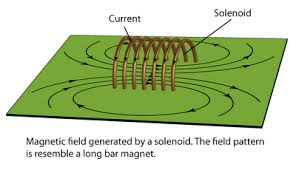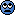# Design & Build Electro-Magnet to Raise 1 KG weight

Hi,
I need to create an electromagnet that can lift a 1 KG Iron block that is kept at 30 cm away from the electromagnet.
I have a DC power supply(RXN 305 D) that can provide a voltage of 0-30 Volt and current of 0-5 A. I need to limit the current used to maximum of 3 Ampere.

Please help to design the core of the electromagnet, the number of turns and other factors to consider to achieve the goal above.

The inspiration behind this is that-
I had seen a youtube video recently where a person produce a strong magnet which could lift 50Lbs using the current from a simple 1.5 volt battery. I tried to wind the insulated copper wire in a normal iron nail we find in house ( 3 rounds one above the other cover complete needle) and passed up to 3 A current through it, but the magnet was not powerful like I found in the video. So I am trying to understand what could be done to make a powerful magnet like the one found in the video.

Hesch
Gold Member
I need to create an electromagnet that can lift a 1 KG Iron block that is kept at 30 cm away from the electromagnet.
You should use a core with the same shape as in the video: E. A solenoid will not do the job.

The cross section areas of the core/load are important: The bigger, the better.

The lifting force is due to the magnetic energy density in the airgap between magnet/load:

Edens = ½ * B * H [ J/m3 ] = B2 / 2μ0 [ J/m3 ]

E = Edens * Volume = Edens * A * s ( A = cross section area , s = distance ).

F = dE/ds

From the above you can calculate the needed B-field ( or the needed H-field ) to lift the load.

From the needed H-field, you can calculate the number of windings and current required, using Amperes law:

circulationH⋅ds = N * I

Forget a distance as of 30cm ( you will never make it work, unless using a cross section area = 1 m2).

EDIT: In the video the magnet can lift about 23kg ( supplied by a battery ). I think it can lift about 300kg, supplied with more power ( assuming some dimensions from the video ).

Last edited:
•wetel
Hi,
. Thank you so much for the details given but I couldn't understand many things from it. Could you please explain these formula in words. I am not really getting the concept and what the integral means in the formula. Again why is it not possible to get a distance of 30 cm? Please provide an explanation.

Hesch
Gold Member
why is it not possible to get a distance of 30 cm?
That's because the magnetic fields will "spread out" at the ends of the solenoid:A magnetic field consists of a H-field and a B-field. In air ( in the airgap ) the relation will be: B = μ0*H → H = B / μ0 , μ0 = 4E-7*π.
In iron the relation will be: B = μ0μr*H , μr ≈ 1000 ( relative permeability ). The electrical analogy is that H corresponds to voltage and that B corresponds to current: I = V / R → V = R*I , R corresponds to 1 / (μ0μr).

Say that at a distance = 30 cm, the field has been weakened a factor 0.1, then the magnetic energy density ( and thus the attracting force ) has been weakened by a factor 0.01. Closing up a permanent magnet to some iron, you can sense it.
I am not really getting the concept and what the integral means in the formula.
The circulation integral is Amperes law. It states that integrating along a closed loop, surrounding N wires conducting a current I, the mean strength of H will be N*I/s , where s is the length of the integration path. So when the path goes through iron all the way, the calculation is quite simple.

In the iron core, a max magnetic induction ( B-field ) of ≈1.4T can be provided. So you must convert this B to H and by means of Amperes law, the N*I can be calculated.

Say that at a distance = 30 cm, the field has been weakened a factor 0.1, then the magnetic energy density ( and thus the attracting force ) has been weakened by a factor 0.01. Closing up a permanent magnet to some iron, you can sense it.

If i need to ensure that a strong magnetic field is available even at a distance of 30 cm, what size of Iron core should I use, How many turns of wire and how many amperes of current needs to be used?

The circulation integral is Amperes law. It states that integrating along a closed loop, surrounding N wires conducting a current I, the mean strength of H will be N*I/s , where s is the length of the integration path. So when the path goes through iron all the way, the calculation is quite simple.

How to percieve this H field and B field. Exaclty what is it? I mean how to percieve it with any of our 5 senses? Also, about this integration thing, I am not so good at it. What is meant by length of integration path and why is it chosen that way?

mfb
Mentor
I mean how to percieve it with any of our 5 senses?
A magnetic field? Not at all (for realistic fields), you need some tool to measure it (and display so your eye can read off the value), like a Hall probe for example.
If i need to ensure that a strong magnetic field is available even at a distance of 30 cm, what size of Iron core should I use
See post 2. Forget it. Such a magnet would be extremely dangerous as well, and should not be built or used by someone without the necessary experience to hande it safely.

A magnetic field? Not at all (for realistic fields), you need some tool to measure it (and display so your eye can read off the value), like a Hall probe for example.

No. I mean the H field and B field- What is it? How to percieve it.

[QUOTE="See post 2. Forget it. Such a magnet would be extremely dangerous as well, and should not be built or used by someone without the necessary experience to hande it safely.[/QUOTE]
Why is it unsafe? But I would like to build one for an experiment. Please help. If i need to ensure that a strong magnetic field is available even at a distance of 30 cm, what size of Iron core should I use, How many turns of wire and how many amperes of current needs to be used?

Last edited:
mfb
Mentor
No. I mean the H field and B field- What is it?
A magnetic field. B-field and H-field are two different ways to quantify its strength.
Why is it unsafe?
Flying metallic objects at high speeds and bruises are dangerous.
If i need to ensure that a strong magnetic field is available even at a distance of 30 cm, what size of Iron core should I use, How many turns of wire and how many amperes of current needs to be used?
See post 2 and 4. If those don't help, you really should not build such a thing.

The lifting force is due to the magnetic energy density in the airgap between magnet/load:

Edens = ½ * B * H [ J/m3 ] = B2 / 2μ0 [ J/m3 ]

E = Edens * Volume = Edens * A * s ( A = cross section area , s = distance ).

F = dE/ds

From the above you can calculate the needed B-field ( or the needed H-field ) to lift the load

What is J/m3 ? Is it Joules per cubic metre?

in E- Edens*Volume, volume of what? and A is cross Section area of what? and S is distance from what to what? Please clarify this

Hesch
Gold Member
I mean the H field and B field- What is it? How to percieve it.
The H-field could by an analogy be compared to an electric field strength [ V/m ].
The B-field could be compared to current density → flux could be compared to current.
What is J/m3 ? Is it Joules per cubic metre?
Yes.
in E- Edens*Volume, volume of what?
Volume of the airgap.
A is cross Section area of what?
The Cross section area of the airgap.
S is distance from what to what?
Distance between magnet and load (height of the airgap)

I have attached the file, elm.pdf :
Here a magnet core is sketched. The distance from the core to the load is 30cm. The green lines illustrate the flux through the airgap and have a total length = 2*30cm = 60cm. Now, if the distance between the legs of the core is shorter than 60cm, the flux will choose the shorter airgap, illustrated by the red lines, which will give no lift to the load. Therefore the distance between the legs is here set to 120cm. So all in all the core will become 320cm wide.

The cross section area, A, is 0.08m2.

Flift = Edens * A →
9.82N = Edens *0.08m2
Edens = 122.8 J/m3 = B2 / 2μ0
B = 0.018T →
H = B / μ0 = 14300 A/m →
N*I = 14300 A/m * 0.06 m = 858 A ( for example 858 windings * 1A. That's about 1km wire ).

Now try to calculate the weight of the magnet and load ( iron + copper + 1kg ). Where will you hang it?

Regard the above as a rough calculation.

Good luck#### Attachments

• elm.pdf
14.4 KB · Views: 429
Last edited:
•Jeff Rosenbury
Now try to calculate the weight of the magnet and load ( iron + copper + 1kg ). Where will you hang it?

Regard the above as a rough calculation.

Good luckExtra credit question: How fast will the 1 Kg load be traveling when it contacts the magnet face?It seems a shame to build something so beefy only to have it smash the first time you use it. Some sort of shock absorber might be in order.

Hesch
Gold Member
Extra credit question: How fast will the 1 Kg load be traveling when it contacts the magnet face?Hearing protector ?